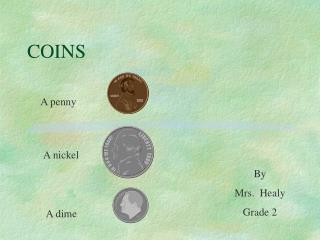DownloadDownload PresentationCOINS

# COINS

Télécharger la présentation## COINS

- - - - - - - - - - - - - - - - - - - - - - - - - - - E N D - - - - - - - - - - - - - - - - - - - - - - - - - - -
##### Presentation Transcript

1. COINS A penny A nickel By Mrs. Healy Grade 2 A dime

2. A penny A penny is worth \$.01.

3. A nickel A nickel is worth \$.05. A nickel is worth 5 pennies.

4. A dime A dime is worth \$.10. A dime is worth 2 nickels. A dime is worth 10 pennies.

5. What is the total amount? A. \$.03 B. \$.17 C. \$.21

6. The total amount was B. \$.17

7. What is the total amount? A. \$ .24 B \$ .14 C \$ .19

8. The total amount was C. \$.19

9. What is the total amount? A. \$.55 B. \$.47 C. \$.27

10. The total amount was B. \$.47

11. What is the total amount? A. \$.53 B. \$.38 C \$.43

12. The total amount was C. \$.43